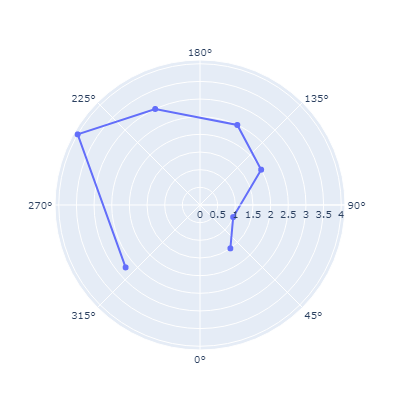# Polar plot with 0° at the nadir?

Is there any simple way to do this? The default is 0° on the right.

Setting in `PlotlyJS.Layout` polar=attr(angularaxis_rotation=270)` does the job, i.e. the origin is rotated 270 degrees:

``````using PlotlyJS
fig1 = Plot(scatterpolar(r = [1.5, 1,2, 2.5, 3, 4, 2.75],
theta = [35, 70, 120, 155, 205, 240, 310],
mode = "markers+lines"),
Layout(font_size=10,
width=400, height=400,
polar=attr(angularaxis_rotation=270)))
``````and for barpolar:

``````r=[3.5, 1.85, 2.5, 4.5, 4, 3.38, 3]
fig2 = Plot(barpolar(r=r,
theta=[65, 15, 210, 110, 312.5, 180, 270],
marker_color=r,
marker_line_color="black", #line around each bar
marker_line_width=0.5),
Layout(font_size=10, width=400, height=400,
polar=attr(angularaxis_rotation=270)))
``````1 Like

Thanks. I was trying to do this with plots and GR, but it doesn’t look like GR supports this (or maybe I can’t find the parameter), so I’ll try your suggestion next.

Yeah, doesn’t seem `GR` supports this.
But this can be forced by digging into Plots.jl.

Try the following code:

Big function here...
``````import Plots: gr_polaraxes

function gr_polaraxes(rmin::Real, rmax::Real, sp::Plots.Subplot)
GR.savestate()
xaxis = sp[:xaxis]
yaxis = sp[:yaxis]

orig_α = 0:45:315
a = orig_α .+ 90
sinf = sind.(a)
cosf = cosd.(a)
α = collect(circshift(orig_α, 2))
rtick_values, rtick_labels = Plots.get_ticks(sp, yaxis, update = false)

# draw angular grid
if xaxis[:grid]
Plots.gr_set_line(
xaxis[:gridlinewidth],
xaxis[:gridstyle],
xaxis[:foreground_color_grid],
sp,
)
Plots.gr_set_transparency(xaxis[:foreground_color_grid], xaxis[:gridalpha])
for i in eachindex(α)
GR.polyline([sinf[i], 0], [cosf[i], 0])
end
end

if yaxis[:grid]
Plots.gr_set_line(
yaxis[:gridlinewidth],
yaxis[:gridstyle],
yaxis[:foreground_color_grid],
sp,
)
Plots.gr_set_transparency(yaxis[:foreground_color_grid], yaxis[:gridalpha])
for i in eachindex(rtick_values)
r = (rtick_values[i] - rmin) / (rmax - rmin)
if r <= 1.0 && r >= 0.0
GR.drawarc(-r, r, -r, r, 0, 359)
end
end
GR.drawarc(-1, 1, -1, 1, 0, 359)
end

# prepare to draw ticks
Plots.gr_set_transparency(1)
GR.setlinecolorind(90)
GR.settextalign(GR.TEXT_HALIGN_CENTER, GR.TEXT_VALIGN_HALF)

# draw angular ticks
if xaxis[:showaxis]
GR.drawarc(-1, 1, -1, 1, 0, 359)
for i in eachindex(α)
x, y = GR.wctondc(1.1 * sinf[i], 1.1 * cosf[i])
GR.textext(x, y, string((360 - α[i]) % 360, "^o"))
end
end

if yaxis[:showaxis]
for i in eachindex(rtick_values)
r = (rtick_values[i] - rmin) / (rmax - rmin)
if r <= 1.0 && r >= 0.0
x, y = GR.wctondc(0.05, r)
Plots.gr_text(x, y, Plots._cycle(rtick_labels, i))
end
end
end
GR.restorestate()
end

``````

This did it on my machine:

``````julia> plot(r, 0, 2π, proj=:polar, lims=(0,1.5));
``````

gives:

Note, the series didn’t really change, only the axis labels, so it might be easiest to transform the data series to fit the new labels (or dig more into the code).

Plots.jl did not intend for this feature to exist… yet.

The changed bit is the:

``````orig_α = 0:45:315
a = orig_α .+ 90

α = collect(circshift(orig_α, 2))
``````

at the beginning.

1 Like

Thanks. On my slightly different function call this draws an error message, unfortunately. I suspect I’ve got the function call wrong.

`plot(deg2rad.(pho.verticalangles), pho.candelavalues[6,:], 0, 2π, proj = :polar, m = 2)`

pho.verticalangles and pho.candelavalues[6,:] are both Vector{Float32} of length 11.

Couldn’t process recipe args: (Vector{Float32}, Vector{Float32}, Int64, Float64)

I’ve gone with the PlotlyJS solution…sure wish I could get it going in IJulia, but it works fine in the basic Julia REPL.

But that is probably another thread.

Try without the `0, 2π`:

``````plot(deg2rad.(pho.verticalangles), pho.candelavalues[6,:]; proj = :polar, m = 2)
``````

Thanks, again!

Your code plus the following command produces the output I was looking for:

`plot(deg2rad.(pho.verticalangles .- 90), pho.candelavalues[6,:], proj = :polar, m = 2)`

1 Like

And I’ve filed a GR enhancement request. It seems like such an obvious thing, and yet…

Has this been implemented now and if so, how to do it?

I don’t know. Back in 2022 I opened an enhancement request and Josef Heinen said it would be picked up in the next release, but I’m not sure that’s occurred yet.

Thank you - I made a follow up comment, to hopefully show more interest in the optionKind regards

Code by @jheinen:

``````using GR
angles = LinRange(0, 2pi, 40)